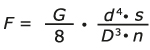# How do you calculate the force of a compression spring?

The force of a compression spring can be calculated using the following formula: